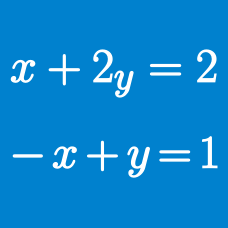Algebra

# System of Equations: Level 4 Challenges

$\dfrac{x}{1-x^2}+\dfrac{y}{1-y^2}+\dfrac{z}{1-z^2} \\ =\dfrac{kxyz}{(1-x^2)(1-y^2)(1-z^2)}$

If $x,y,$ and $z$ are real numbers satisfying $xy+yz+xz=1$, find the value of $k$ for which the equation above holds true.

If $a, b$ and $c$ are non-zero real numbers, that satisfy the equations $a^2 + b^2 + c^2 = 1$ and $a\left(\frac {1}{b} + \frac {1}{c}\right) + b\left(\frac {1}{c} + \frac {1}{a}\right) + c\left( \frac {1}{a} + \frac {1}{b}\right) = -3$, how many possible values are there for $a + b + c$?

How many unordered triplets $(x,y,z)$ are in the range $[0,2]$ and satisfy the system of equations: $\left\{\begin{array}{l}2x^2-4x+2=y\\ 2y^2-4y+2=z\\ 2z^2-4z+2=x\end{array}\right.$

Details and Assumptions:

An unordered triplet means that $(1,2,3)$ is the same as $(3,2,1)$ or $(1,3,2)$.

The positive reals $x,y$ and $z$ satisfy the given equations:

$x^{2}+xy+\frac{y^{2}}{3}=25$

$z^{2}+\frac{y^{2}}{3}=9$

$z^{2}+zx+x^{2}=16$

Find the value of $(xy+2yz+3xz)^{2}$.

$x$ satisfies the equation $\sqrt{x+\sqrt{x}}-\sqrt{x-\sqrt{x}}=\dfrac{199}{100}\sqrt{\dfrac{x}{x+\sqrt{x}}}$

Also, its value can be expressed in the form $\frac{m}{n}$, where $m$ and $n$ are coprime positive integers, find $m+n$.

×

Problem Loading...

Note Loading...

Set Loading...